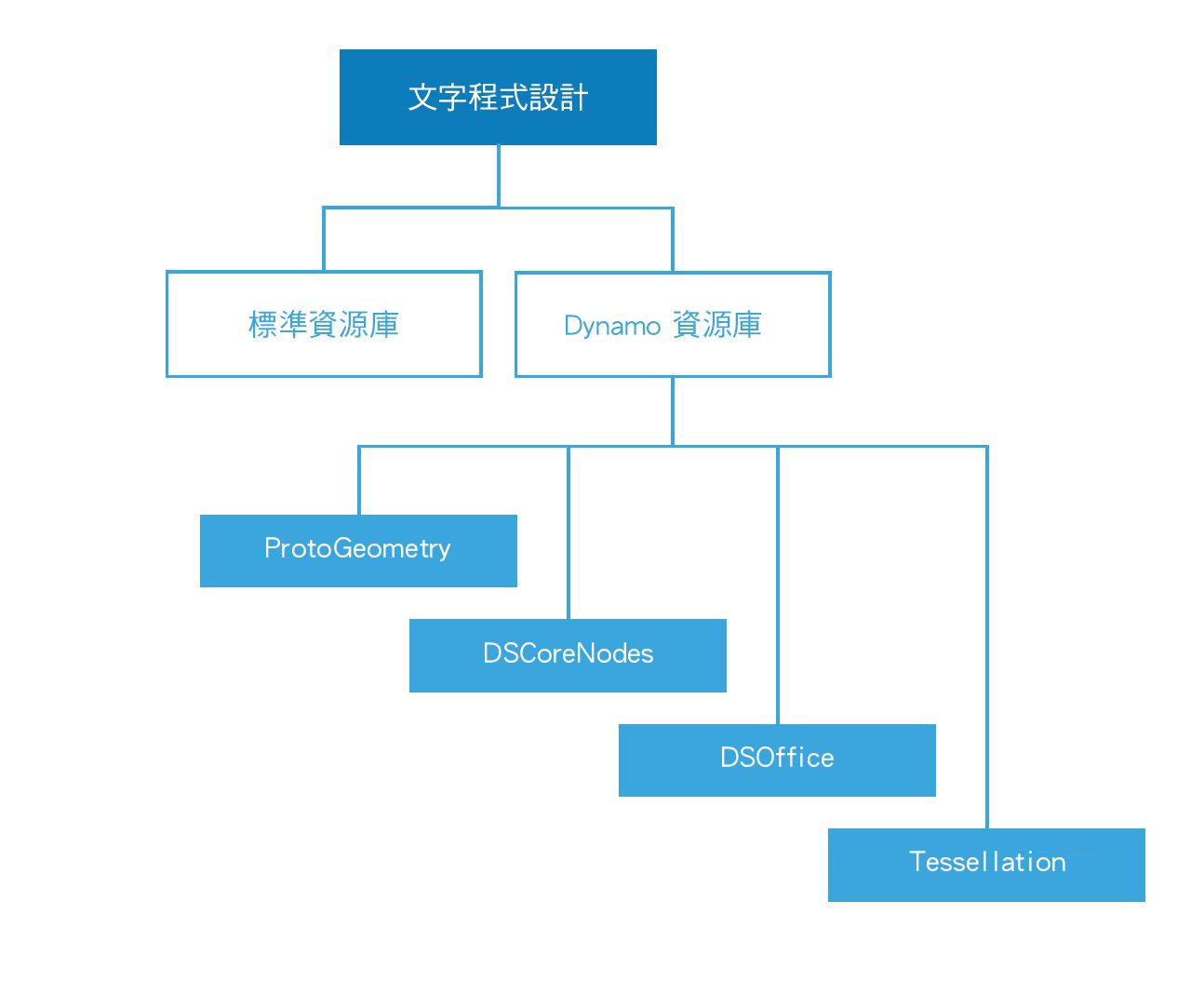## 腳本參考

### 要使用的資源庫• Python 和 C# 的標準資源庫可以用於在 Dynamo 環境中建置進階資料和流動結構。
• Dynamo 資源庫直接對應用於建立幾何圖形和其他 Dynamo 物件的節點架構。

Dynamo 資源庫

• ProtoGeometry
• 功能：弧、邊界框、圓形、圓錐體、座標系統、立方體、曲線、圓柱邊、邊緣、橢圓、橢圓弧、面、幾何圖形、螺旋線、索引群組、線、網面、Nurbs 曲線、Nurbs 曲面、平面、點、多邊形、矩形、實體、圓球、表面、拓樸、T 雲形線、UV、向量、頂點。
• 如何匯入：`匯入 Autodesk.DesignScript.Geometry`
• 注意事項：當透過 Python 或 C# 使用 ProtoGeometry 時，您將建立不受管理的物件，而這需要手動管理記憶體 - 請參閱以下部分：不受管理的物件，以取得更多資訊。

• DSCoreNodes

• 功能：顏色、顏色範圍 2D、日期時間、時間跨距、IO、公式、邏輯、清單、數學、四元樹狀目錄、字串、螺紋。
• 如何匯入：`import DSCore`

• 鑲嵌

• 功能：凸面關聯線，Delaunay、Voronoi。
• 如何匯入：`import Tessellation`

• DSOffice

• 功能：Excel。
• 如何匯入：`import DSOffice`

### 仔細標示

``````### BAD
csfX = 1.6
csfY= 1.3
csfZ = 1.0
``````
``````### GOOD
# column scale factor (csf)
csfX = 1.6
csfY= 1.3
csfZ = 1.0
``````

``````### BAD
import car
seat = car.CarSeat()
tire = car.CarTire()
``````
``````### GOOD
import car
seat = car.Seat()
tire = car.Tire()
``````

``````### BAD
if 'mystring' not in text:
else:
print 'found'
print 'processing'
``````
``````### GOOD
if 'mystring' in text:
print 'found'
print 'processing'
else:
``````

``````### BAD
agents = …
active_agents = …
``````
``````### GOOD
agents = …
agents_active = …
``````

``````### BAD
from RevitServices.Persistence import DocumentManager

DocumentManager = DM

doc = DM.Instance.CurrentDBDocument
uiapp = DM.Instance.CurrentUIApplication
``````
``````### GOOD
from RevitServices.Persistence import DocumentManager as DM

doc = DM.Instance.CurrentDBDocument
uiapp = DM.Instance.CurrentUIApplication
``````

``````### BAD
rotateToCoord = rotateFromCoord.Rotate(solid.ContextCoordinateSystem.Origin,Vector.ByCoordinates(0,0,1),5)
``````
``````### GOOD
toCoord = fromCoord.Rotate(solid.ContextCoordinateSystem.Origin,Vector.ByCoordinates(0,0,1),5)
``````

「一切都應該做得愈簡單愈好，但是不要過於簡單。」– 愛因斯坦 (Albert Einstein)

### 型式一致

• 變數、函數、方法、套件、模組：
`lower_case_with_underscores`

• 類別和例外情況：
`CapWords`

• 受保護的方法和內部函數：
`_single_leading_underscore(self, ...)`

• 私用方法：
`__double_leading_underscore(self, ...)`

• 常數：
`ALL_CAPS_WITH_UNDERSCORES`

• 用兩個空白行包圍頂層函數與類別定義。

• 類別中的方式定義被一個空白行包圍。

• 額外的空白行可用於分隔擁有相關功能的群組 (請斟酌使用)。

• 在括弧、括號或支撐內：
``````### BAD
function( apples[ 1 ], { oranges: 2 } )
``````
``````### GOOD:
function(apples, {oranges: 2})
``````
• 在逗號、分號或冒號前：
``````### BAD
if x == 2 : print x , y ; x , y = y , x
``````
``````### GOOD
if x == 2: print x, y; x, y = y, x
``````
• 在啟動函數呼叫的引數清單之左括弧前方：
``````### BAD
function (1)
``````
``````### GOOD
function(1)
``````
• 在啟動索引或切割之左括弧前方：
``````### BAD
dict ['key'] = list [index]
``````
``````### GOOD
dict['key'] = list[index]
``````
• 始終在這些二進位運算子的兩側加入單個空間:
``````assignment ( = )
augmented assignment ( += , -= etc.)
comparisons ( == , < , > , != , <> , <= , >= , in , not in , is , is not )
Booleans ( and , or , not )
``````

• 不宜過度強調它 ~ 79 個字元。

• 限制所需編輯器視窗寬度可以同時開啟多個檔案，並且適合使用在相鄰的欄顯示兩個版本的程式碼檢閱工具。

• 透過折繞括弧內的表示式，長線可以在多條線上斷開：

• 有時更少的註釋使程式碼更易於讀取，尤其是如果它強制您使用有意義的符號名稱。

• 良好的編碼可減少依賴註釋：

``````### BAD
# get the country code

# if country code is US
if (country_code == 'US'):
# display the form input for state
print form_input_state()
``````
``````### GOOD
# display state selection for US users
if (country_code == 'US'):
print form_input_state()
``````

• 開放源碼專案由大量開發人員協同合作建置。這些專案需要保持高度的程式碼可讀性，以便團隊可以盡可能地有效工作。因此，最好瀏覽這些專案的來源代碼，以觀察這些開發人員的作業。

• 改善慣例：

• 詢問自己，每個慣例是否能回應目前工作的需求。

• 功能/效率有否受到影響？

### C# (Zerotouch) 標準

``````public Cuboid singleCube(){

var output = Cuboid.ByLengths(1,1,1);

for(int i = 0; i<10000;i++){
output = Cuboid.ByLengths(1,1,1);
}
return output;
}
``````

``````public Cuboid singleCube(){

var output = Cuboid.ByLengths(1,1,1);
var toDispose = new List<Geometry>();

for(int i = 0; i<10000;i++){